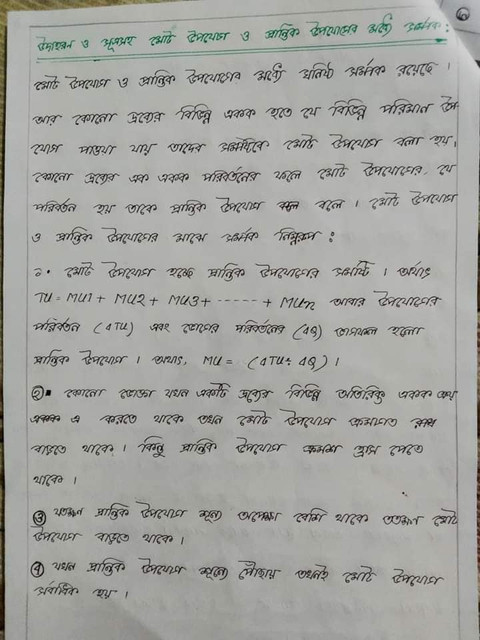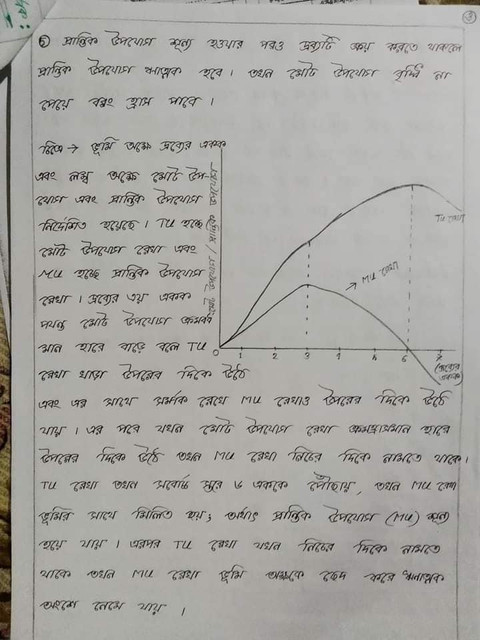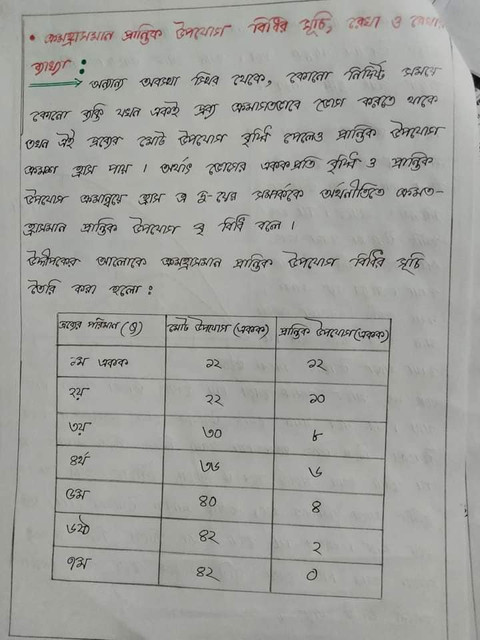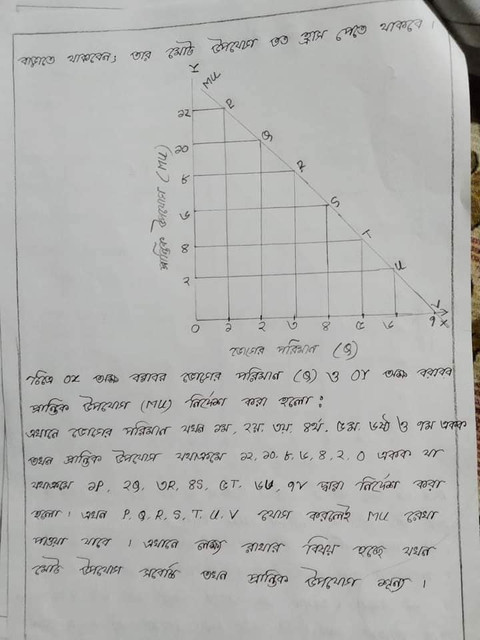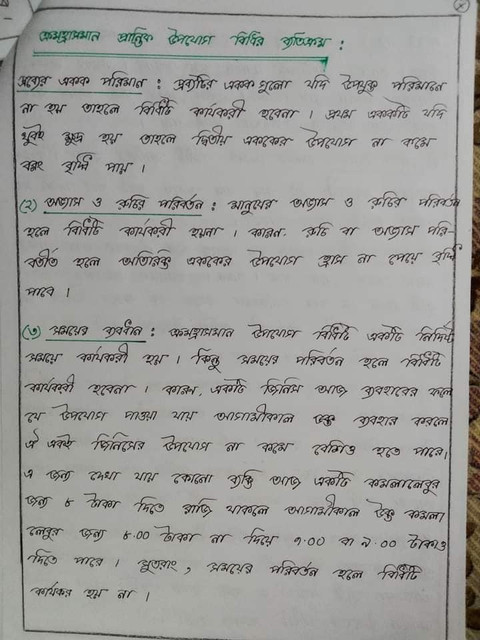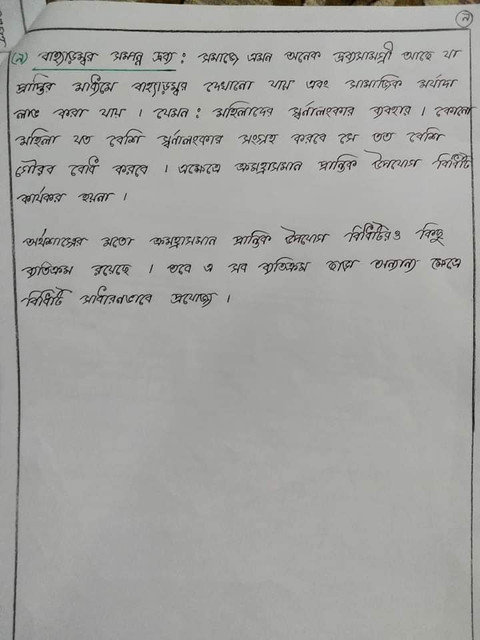Assignment

# Hsc 2023 1st Weak Economics Assignment Answer

The first assignment for the first week of the 2023 HSC exam assignment economics textbook for students choosing economics as an optional subject in the humanities department has been taken from the second chapter: From the Behavior of the Producer.

After completing the first assignment in Economics No. 17, the students of the humanities department will be able to explain the concept of utility, determine the relationship between total and initial utility, and draw a chart of declining marginal utility rules based on hypothetical schedule / real events.

## Hsc 2023 1st Weak  Assignment Economics

The picture below mentions the details of HSC 2023 Economics First Paper 1st Assignment (HSC 2023 Economics First Paper 1st Assignment).Level: HSC Exam 2023, Category: Humanities, Subject: Economics, Paper: First, Subject Code-109, Assignment No-1

Chapter and Title: Chapter Two: Producer’s Behavior.

Assignment: In the case of the first, third, fifth and seventh unit divisions of a consumer mate মা the first, third, fifth and seventh unit divisions have 12, 30, 40 and 42 units respectively and the second, fourth and sixth unit divisions have marginal applications of 10, 6 and 2 units respectively.

Submitting the relevant rules of the textbook with the help of diagrams with exceptions subject to preparation of complete index using the given information;

Learning Outcomes / Contents: Explain the concept of application; Be able to determine the relationship between MAT and marginal use; I will be able to explain it by drawing a chart of declining marginal usage rules on the basis of hypothetical schedule / real events.

Instructions (signal / step / circumference):

1. Concept of utility;

2. The relationship between met utilization and marginal utilization;

3. Declining marginal usage rules;

4. Exceptions to the declining marginal use rules;

## Hsc 2023 1st Weak Economics Assignment Answer